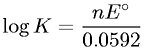Equations > Chemistry > Electrochemistry > Relationship between Equilibrium Constant and Cell Voltage

### Relationship between Equilibrium Constant and Cell VoltageLatex Code:

MathML Code:

 $\mathrm{log}K=\frac{\mathrm{nE}}^{\circ }0.0592$

MathType 5.0: